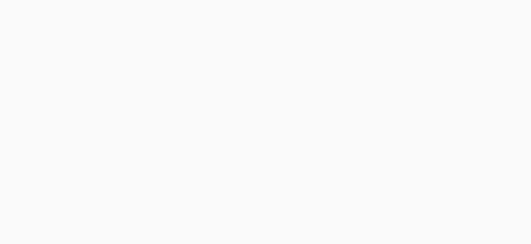#Quants Questions : Data Interpretation Set 15

Hello Aspirants. Welcome to Online Quantitative Aptitude section in AffairsCloud.com. Here we are creating question sample From Data Intrepretation that is important for IBPS,SBI exam,SSC exam, Railways Exam,FCI exam  and other competitive exams. We have included Some questions that are repeatedly asked in exams !!

I. Study the following information to answer the given questions1. What is the ratio of total number of staff in ECE,EEE to the total no of students in CSE and IT ?
A)14:1
B)1:14
C)1:12
D)1:17
E)None of these
B)1:14
Explanation :
Staff = 200
Students = 2800
200: 2800 = 2:28 = 1:14

2. The total no of male staff in IT and EIE is what % of the total number of staff in these 2 dept ?
A)44.5%
B)45.7%
C)47.5%
D)45.00%
E)None of these
C)47.5%
Explanation :
IT = 90 => 4:5 => 40(male)
EIE = 70 => 18:17 => 36(male)
40+36 = 76
Total = 90+70 = 160
76*100/160 = 47.5%

3. Total no of female students in CSE is what % more than the male students in the same Dept ?
A)44.45%
B)40.76%
C)40.75%
D)40.66%
E)None of these
A)44.45%
Explanation :
2200 => 9:13 => 900:1300
400*100/900 = 44.45%

4. Find the number of female students in ME dept ?
A)850
B)650
C)150
D)750
E)None of these
D)750
Explanation :1600 => 17:15 = 850 : 750(f)

5. Find the average of total no of staff in all the dept ?
A)103.8
B)108.3
C)108.2
D)108
E)None of these
B)108.3
Explanation :(120+80+150+90+140+70)/6 = 650/6 = 108.3

II. As  part of renovation of the home, flooring, painting and carpeting of a hall is to be undertaken. The hall has length 10m, breath 12m and height 5m. The cost of flooring is Rs.2500perm2 , painting is Rs.600 per sq m and carpeting is Rs.500 per sq m

1. What will be the cost of carpeting the hall leaving aside the area of 10m *4.5m for the wooden sofa?
A)37,500
B)35,700
C)35,500
D)34,500
E)None of these
A)37,500
Explanation :500(10*12 – 10*4.5) = 500*75 = 37,500

2. What will be the cost of flooring the house if the contractor offered 10% discount on the total cost ?
A)2,70,300
B)3,00,000
C)3,30,000
D)2,70,000
E)None of these
D)2,70,000
Explanation :
10*12*2500 = 3,00,000
3,00,000*10/100 = 30,000
3,00,000 – 30,000 = 2,70,000

3. What will be the total cost of flooring and painting the Ceiling ?
A) 3,72,000
B) 3,70,000
C) 3,27,000
D) 3,00,000
E)None of these
A) 3,72,000
Explanation :10*12*2500 + 10*12*600 = 3,00,000 + 72,000 = 3,72,000

4. What will be the difference between the cost of painting the house on length side and the wall on the breath side provided neither of the walls has a door or a window
A)5000
B)4000
C)6000
D)6400
E)None of these
C)6000
Explanation :
12*5- 10*5  = 60 – 50 = 10
10*600 = 6000

5. If the cost of the plastering is Rs.350 then what will be the cost of the plastering the wall ?
A)16,500
B)17,000
C)17,250
D)17,500
E)None of these
D)17,500
Explanation :
10*5 = 50
50*350 = 17,500

AffairsCloud Ebook - Support Us to Grow